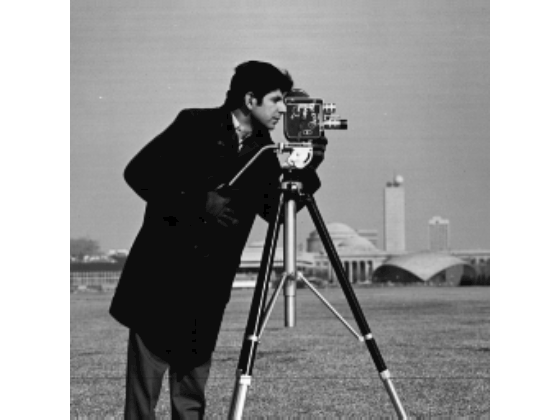denoiseImage

Denoise image using deep neural network

Description

example

B = denoiseImage(A,net) estimates denoised image B from noisy image A using a denoising deep neural network specified by net.

This function requires that you have Deep Learning Toolbox™.

Examples

collapse all

Load the pretrained denoising convolutional neural network, 'DnCNN'.

net = denoisingNetwork('DnCNN');

Load a grayscale image into the workspace, then create a noisy version of the image.

noisyI = imnoise(I,'gaussian',0,0.01);

Display the two images as a montage.

montage({I,noisyI})
title('Original Image (Left) and Noisy Image (Right)')Remove noise from the noisy image, then display the result.

denoisedI = denoiseImage(noisyI,net);
imshow(denoisedI)
title('Denoised Image')Input Arguments

collapse all

Noisy image, specified as a single 2-D image or a stack of 2-D images. A can be:

• A 2-D grayscale image with size m-by-n.

• A 2-D multichannel image with size m-by-n-by-c, where c is the number of image channels. For example, c is 3 for RGB images, and 4 for four-channel images such as RGB images with an infrared channel.

• A stack of equally-sized 2-D images. In this case, A has size m-by-n-by-c-by-p, where p is the number of images in the stack.

Data Types: single | double | uint8 | uint16

Denoising deep neural network, specified as a SeriesNetwork (Deep Learning Toolbox) object. The network should be trained to handle images with the same channel format as A.

Output Arguments

collapse all

Denoised image, returned as a single 2-D image or a stack of 2-D images. B has the same size and data type as A.